# The Nets of Three Dimensional Shapes (Prisms and Pyramids)

#### LESSON – THE NETS OF THREE DIMENSIONAL SHAPES

###### TOPIC
• Introduction
• Nets of Prisms
• Net of Triangular Prism
• Net of Square Prism
• Net of Rectangular Pyramid
• Net of Triangular Pyramid
• Net of Square Pyramid
• Net of Rectangular Pyramid

###### INTRODUCTION

A net is a pattern made from two dimensional shape to form three dimensional shape.

###### THE NETS OF PRISM

The net of a prism consists of six faces.

Focus prisms are triangular, square and rectangular prisms.

###### NET OF TRIANGULAR PRISM

The net of a triangular prism consists of two triangle with three squares or rectangles.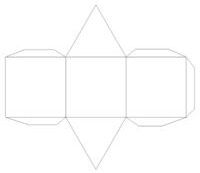###### NET OF SQUARE PRISM

The net of a square prism consists of six squares.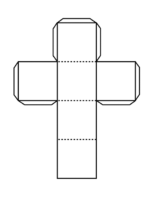###### NET RECTANGULAR PRISM

The net of a rectangular prism consists of two squares and four rectangles.

Rectangular prism is also as cuboid.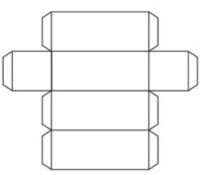###### MAKING OF PRISM

Materials Needed are cardboard, pencil, ruler and gum.

1. Draw the nets of cube and cuboid.

2. Use the nets to make cube and cuboid.

###### NET OF SQUARE PYRAMID

The net of a square pyramid consists of one square and four triangles.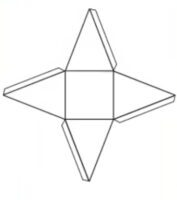###### NET OF TRIANGULAR PYRAMID

The net of a triangles pyramid consists of four triangles.

One of the triangle is a base.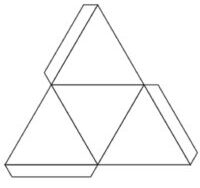###### NET OF RECTANGULAR BASE PYRAMIDS

The net of a rectangular pyramid consists of one rectangle and four triangles.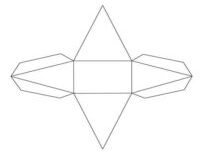###### MAKING OF PYRAMIDS

Materials Needed are cardboard, pencil, ruler and gum.

1. Draw the nets of square, triangular and rectangular pyramids.

2. Use the nets to make square, triangular and rectangular pyramids.

###### NET OF A CONE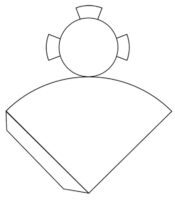###### NET OF A CYLINDER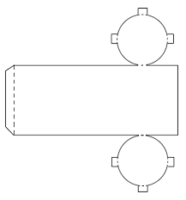###### LESSON EVALUATION

1. explain the meaning of 3 dimensional shapes.

2. differentiate between 2D and 3D shapes.

3. state the uses of three dimensional shapes.

4. state the properties of the following shapes,

• Cube
• Cuboid
• Triangular prism
• Cone
• Sphere
• Pyramid
• Cylinder

5. draw the net of the following:

• Square, triangular and rectangular prisms
• Square, triangular and rectangular pyramids

6. construct prism and pyramid.